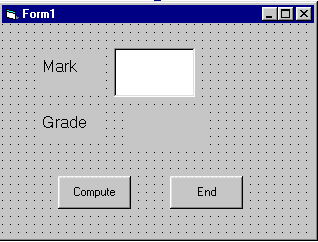# Lesson 14 : Functions and Sub Procedure

### 14.1 Creating Your Own Function

If you wish to create a function that is applicable to the whole project, the syntax is as follows:

``` Public Function functionName(Arg As dataType,.......) As dataType
```

If you wish to create a function that is applicable to a module or a sub procedure, you need to use the keyword Private, as follows:

``` Private Function functionName(Arg As dataType,.......) As dataType
```

#### Example 14.1

In this example, a user can calculate the future value of a certain amount of money he has today based on the interest rate and the number of years from now, supposing he will invest this amount of money somewhere .The calculation is based on the compound interest rate.

#### The code

```Public Function FV(PV As Variant, i As Variant, n As Variant) As Variant
'Formula to calculate Future Value(FV)
'PV denotes Present Value
FV = PV * (1 + i / 100) ^ n
End Function

Private Sub compute_Click()
'This procedure will calculate Future Value
Dim FutureVal As Variant
Dim PresentVal As Variant
Dim interest As Variant
Dim period As Variant
PresentVal = PV.Text
interest = rate.Text
period = years.Text
'calling the function
FutureVal = FV(PresentVal, interest, period)
MsgBox ("The Future Value is" & FutureVal)
End Sub
```#### Example 14.2

The following program will automatically compute examination grades based on the marks that a student obtained. The code is shown below:

#### The Code

```Public Function grade(mark As Variant) As String
Select Case mark
Case Is >= 80
Case Is>= 70
Case Is >= 60
Case Is >= 50
Case Is >= 40
Case Else
End Select
End Function ```
```Private Sub compute_Click()
End Sub```## 14.2 Creating Sub Procedure

A sub procedure(also call subroutine) is a procedure that is called from the main procedure to perform a specific task. It is different from function in the sense that it does not return a value as a function does.A sub procedure is usually used to accept input from the user, display information, print information, manipulate properties or perform some other tasks. It is a program code by itself and it is not an event procedure because it is not associated with a runtime procedure . It is called by other code whenever it is required to perform a certain task. Sub procedures help to make programs smaller and seamless to manage. A sub procedure begins with a Sub ProcedureName keyword and ends with an End Sub keyword.

The structure of a sub procedure is as follows:

```Sub ProcedureName (arguments)

Statements

End Sub
```

#### Example 14.3

In this example, we create a sub procedure to sum up two values that are specified by the arguments . The main program can reference a procedure by using its name together with the arguments in the parentheses.

```Private Sub cmdCal_Click()
Dim x As Single, y As Single
x = Val(TxtNum1.Text)
y = Val(TxtNum2.Text)
sum x, y

End Sub

Sub sum(a As Single, b As Single)
MsgBox ("sum=" & a + b)
End Sub
```

Running the program produces a message boxes as shown in Figure 14.3a and Figure 14.3b#### Figure 14.3a#### Example 14.3b

This program determines buying decision based on shoe' size and its price. In this program, we create a sub procedure known as buy_decision that has two arguments, size and price. A boolean variable buy is included to help in decision making. It takes a value of true or false. If the size and price entered fufilled the requirement via the If..Then...Else statement, buy is assigned a value of true, else it is assigned a value of false.

```Public Sub buy_decision(size As Integer, price As Single)
If size >= 7 And price <= 200 Then
End If

End Sub

Private Sub CmdDecide_Click()
Dim shoe_size As Integer, shoe_price As Single
shoe_size = TxtX.Text
shoe_price = TxtY.Text
End Sub```

#### The Output#### Figure 14.4a

The Output after pressing the buy decision button